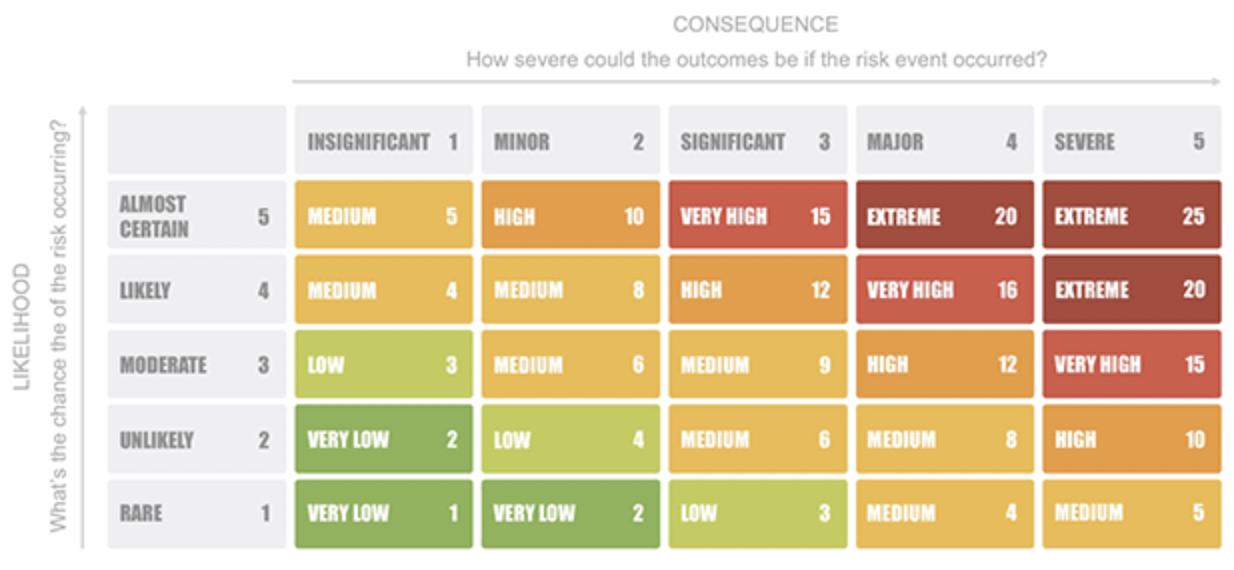Title Page

• Site conducted

• Activity Assessed

• Issue number

• Purpose of new issue

• Add detailed description of review

• Location
• Assessment Date

• Review Date

• Assessor (name)

• Assisted by (names)

Hazard List

• What is the source of Hazard?

• Who is exposed?

• What is the type of injury that could occur?

• What are the existing controls?

• How much is the likelihood of occurrence of hazard

• What could be the severity of Injury/ Disease

• Use the Risk Matrix to determine the risk rating for this hazard• What is the risk rating for this hazard?

• Use the Risk Matrix to determine the risk rating for this hazard• What is the risk rating for this hazard?

• Use the Risk Matrix to determine the risk rating for this hazard• What is the risk rating for this hazard?

• Use the Risk Matrix to determine the risk rating for this hazard

• Use the Risk Matrix to determine the risk rating for this hazard• Use the Risk Matrix to determine the risk rating for this hazard• What is the risk rating for this hazard?

• please first go and complete the risk assessment for COSHH/ handling hazardous materials and ensure you have a current safety data sheet available

• Source of Hazard

• Who is exposed

• What is the type of injury that could occur?

• Existing Controls

• How much is the likelihood of occurrence of hazard

• What could be the severity of Injury/ Disease

• Use the Risk Matrix to determine the risk rating for this hazard• What is the risk rating for this hazard?

• Use the Risk Matrix to determine the risk rating for this hazard• What is the risk rating for this hazard?

• Use the Risk Matrix to determine the risk rating for this hazard• What is the risk rating for this hazard?

• Use the Risk Matrix to determine the risk rating for this hazard• What is the risk rating for this hazard?

• Use the Risk Matrix to determine the risk rating for this hazard• What is the risk rating for this hazard?

• please first complete the risk assessment for Manual handling

• Source of Hazard

• Who is exposed

• What is the type of injury that could occur?

• Existing Controls

• How much is the likelihood of occurrence of hazard

• What could be the severity of Injury/ Disease

• Use the Risk Matrix to determine the risk rating for this hazard• What is the risk rating for this hazard?

• Use the Risk Matrix to determine the risk rating for this hazard• What is the risk rating for this hazard?

• Use the Risk Matrix to determine the risk rating for this hazard• What is the risk rating for this hazard?

• Use the Risk Matrix to determine the risk rating for this hazard• What is the risk rating for this hazard?

• Use the Risk Matrix to determine the risk rating for this hazard• What is the risk rating for this hazard?

• please first go and complete the risk assessment for using display screen equipment (DSE)

• Source of Hazard

• Who is exposed

• What is the type of injury that could occur?

• Existing Controls

• How much is the likelihood of occurrence of hazard

• What could be the severity of Injury/ Disease

• Use the Risk Matrix to determine the risk rating for this hazard• What is the risk rating for this hazard?

• Use the Risk Matrix to determine the risk rating for this hazard• What is the risk rating for this hazard?

• Use the Risk Matrix to determine the risk rating for this hazard• What is the risk rating for this hazard?

• Use the Risk Matrix to determine the risk rating for this hazard• What is the risk rating for this hazard?

• Use the Risk Matrix to determine the risk rating for this hazard• What is the risk rating for this hazard?

• Other Hazard

• Source of Hazard

• Who is exposed

• What is the type of injury that could occur?

• Existing Controls

• How much is the likelihood of occurrence of hazard

• What could be the severity of Injury/ Disease

• Use the Risk Matrix to determine the risk rating for this hazard• What is the risk rating for this hazard?

• Use the Risk Matrix to determine the risk rating for this hazard• What is the risk rating for this hazard?

• Use the Risk Matrix to determine the risk rating for this hazard• What is the risk rating for this hazard?

• Use the Risk Matrix to determine the risk rating for this hazard• What is the risk rating for this hazard?

• Use the Risk Matrix to determine the risk rating for this hazard• What is the risk rating for this hazard?

Control Measures and Personal Protective Equipment Required

• Are machinery protection devices (guards, interlocks) used as a control measure for this task?

• Is there a specific system of work (e.g. SSW, JSA, Work at Height Permit) required for this task?

• Is Hearing Protection required for this task?

• Is Eye Protection required for this task?

• Is Respiratory Protection required for this task?

• Is Hand Protection required for this task?

• Is protective coveralls/ clothing required for this task?

• Is Hi Vis Clothing required for this task?

• Is safety footwear required for this task?

• Is there other personal protective equipment required for this task?